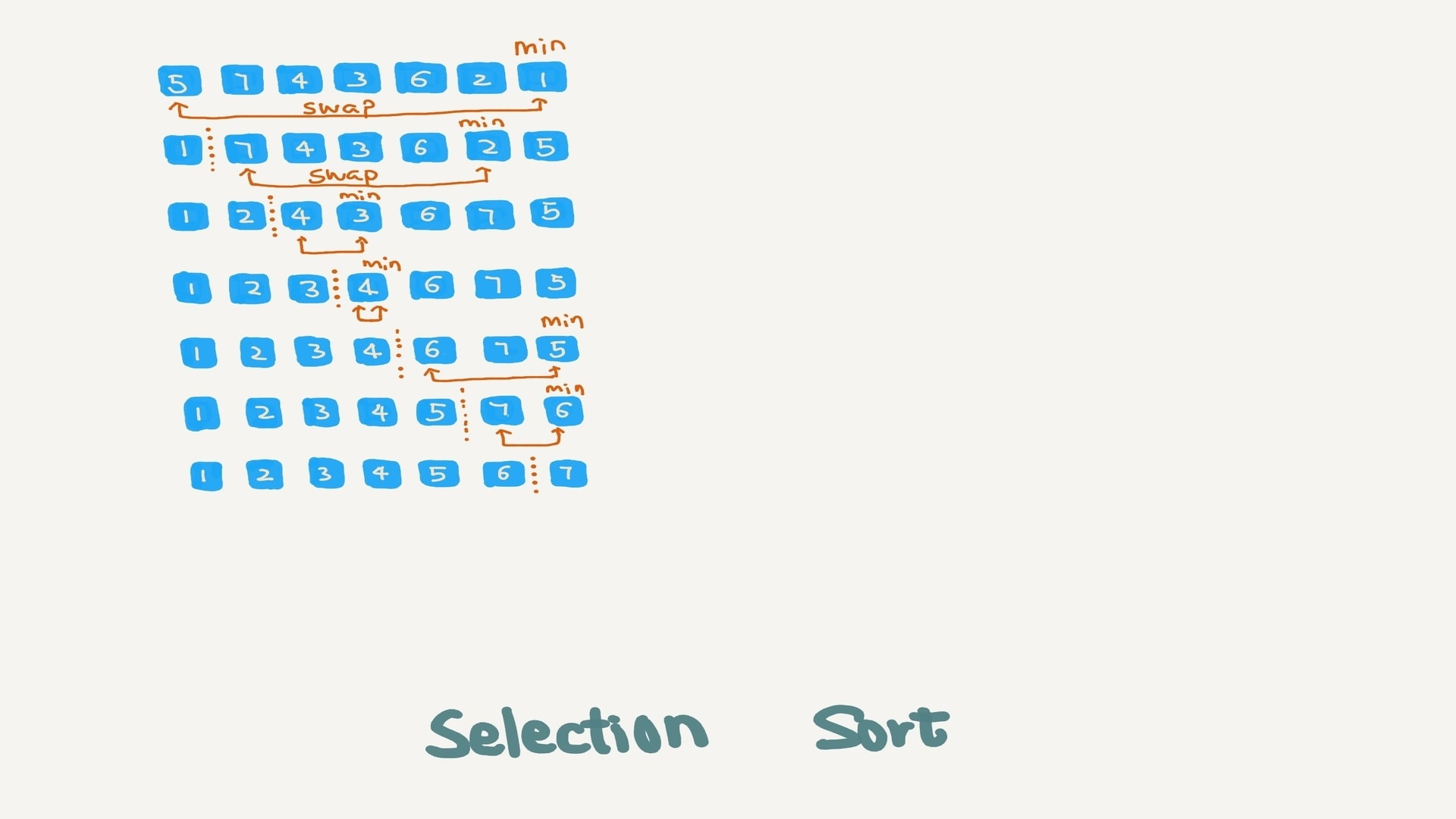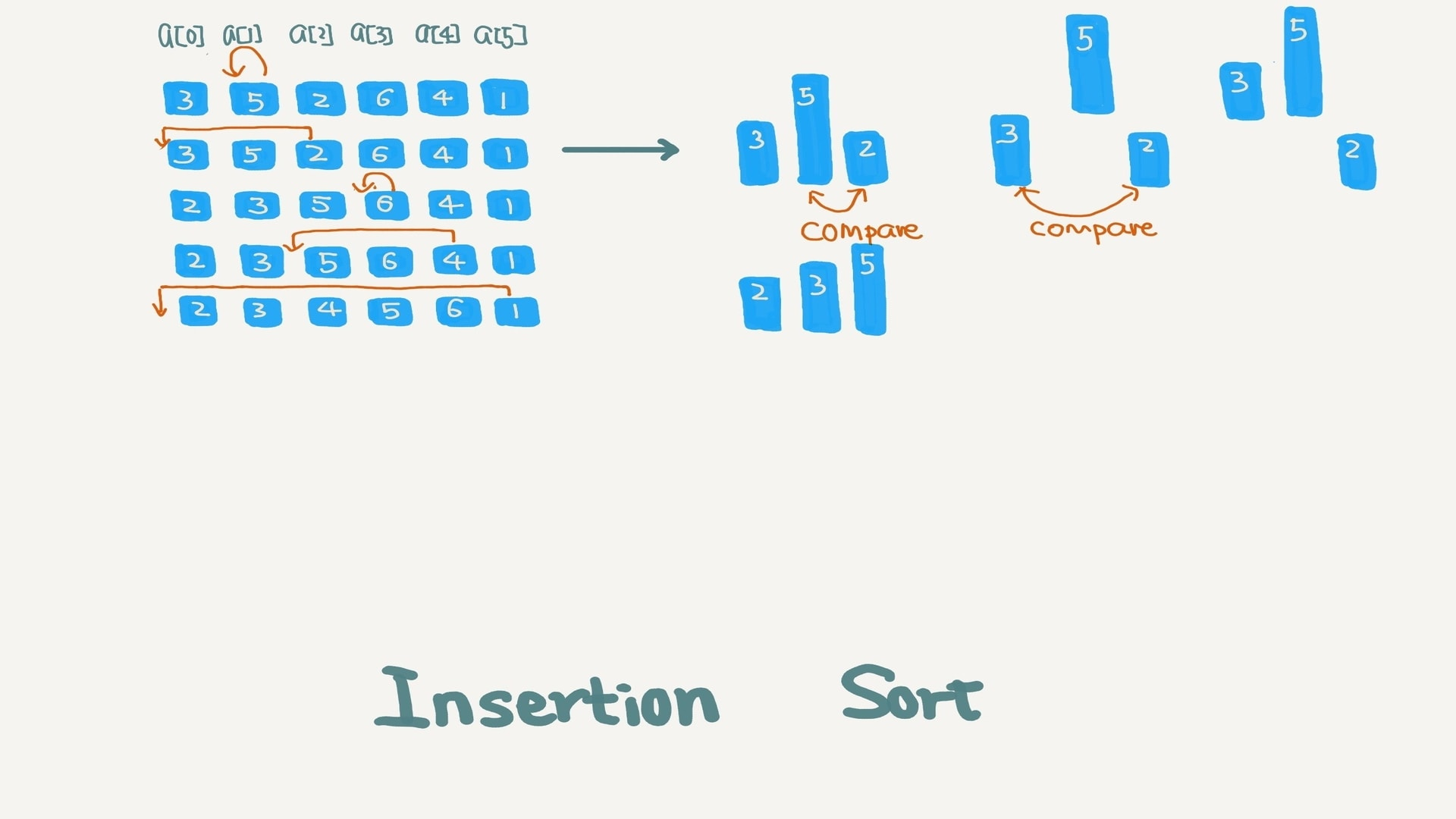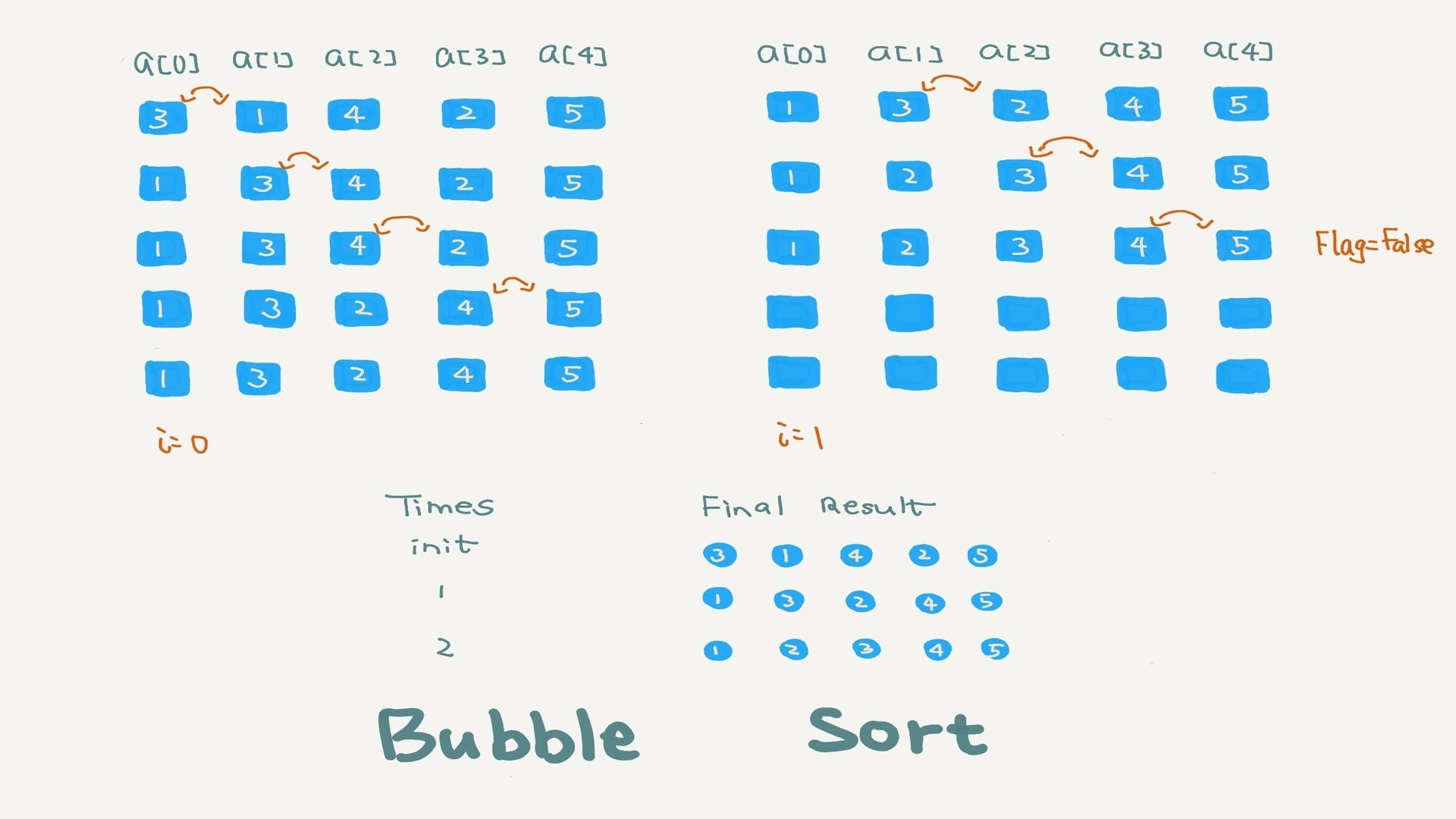# Data Structure and Algorithm Notes (1)

The farther backward you can look, the farther forward you will see.

Winston Churchill

### Why do we need computational complexity analysis?

• #### The limitation of post-hoc analysis

1. The performance will vary according to different hardwares.
2. The size of the data or the way the data is structured will also impact the performance.
• #### Big O notation

On the contrary, the complexity analysis is platform-independent. There are two ways of doing complexity analysis, which are time complexity and space complexity. Big O notation, Big-omega notation and Big-theta notation are used to estimate the complexity function for arbitrarily large input. In this notes, I am only use the Big O notation to analysis the algorithm.
$$\mathcal{T}(n)=\mathcal{O}({f}(n))$$

### Basic Algorithm

• #### Sort

For the code of each kind of sorting algorithm, I kept in my github python repo
1. ##### Selection Sort2. ##### Insertion Sort3. ##### Bubble Sort